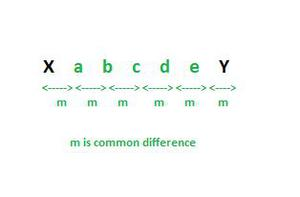Find 5 arithmetic means between two numbers

• Last Updated : 10 Nov, 2021

An arithmetic Sequence can be defined as a sequence of numbers that are in some kind of order or following a pattern. In an Arithmetic sequence, there is some common difference between any two adjacent numbers and that difference is the key of that sequence. Let’s understand with an example, Sequence: 100, 200, 300, 400, 500, 600.

Common Difference

Now in the above sequence, there is some kind of pattern, which means one can guess the next number by seeing the current sequence. Here, the common difference “d” can be found. The common difference is the difference obtained by subtracting a term by its next term.

Common difference (d) = Difference between two adjacent numbers

(Here lets take 100 and 200)

d  = Current number – Previous number

d  = 200 – 100

d  = 100

So now that the common difference d is known, every number in the sequence can be found by following pattern as,

First number = 100

Second number = 100 + d  = 100 + 100 = 200

Third number =100 + 2d = 100 + 2 × 100 = 300

Fourth number = 100 + 3d = 100 + 3 × 100 = 400

Fifth number =100 + 4d = 100 + 4 × 100 = 500

So by following the pattern we can write a general sequence as:

{first number, first number + d, first number + 2d, first number + 3d … and so on}

Let’s take the first number as a and make a general equation for finding the nth number of the sequence. Let’s take the nth number  as an, So the general equation will be:

an= a + (n – 1)d

Here, a = first number

d = common difference

n = total number of terms

an = nth term of the sequence

Arithmetic Mean

Arithmetic mean also known as Average can be defined as the sum of all quantities/values/observations divided by the total number of quantities/values/observations. For example, below are 10 values given,

So arithmetic mean of these values can be calculated as,

Mean = (Sum of all values)/Total number of values

= (2 + 3 + 5 + 8 + 9 + 11 + 13 + 17 + 22 + 10)/10

= (100)/10

= 10

Find 5 arithmetic means between two numbers.

Suppose there are two numbers X and Y. The task is to insert 5 arithmetic means between these two numbers. First of all, let’s visualize that after inserting 5 arithmetic means there are a total of 7 numbers which are in Arithmetic Progression (sequence of numbers in which there is a common difference between any two adjacent numbers).As known that the resulting sequence is an Arithmetic Progression. So, the formula for finding the nth term in the sequence:

an  = a1 + (n-1)d

an = nth term in the sequence

a1 = first term in the sequence

d  = common difference between terms

Now to insert 5 Arithmetic means we use the following method:

Find the common difference d (In above image it is m)

It is clear that if adding common difference m in X will give a,

X + m = a

a + m = b

b + m = c

c + m = d

d + m = e

e + m = Y

So, X + 6m = Y

m = (Y – X)/6

The above equation can be directly obtained using formula, an  = a1 + (n – 1)d

Y = X + (7 – 1)m

As total terms are 7, n is 7

m= (Y – X)/6

Sample Problems

Question 1: Insert 5 Arithmetic Mean between 5 and 23.

Solution:

Find common difference m

X = 5  Y = 23

m = (Y – X)/6

m = (23 – 5)/6

m = 3

Now,  a = X + m = 5 + 3  = 8

b = a + m = 8 + 3  = 11

c = b + m = 11 + 3 = 14

d = c + m = 14 + 3 = 17

e = d + m = 17 + 3 = 21

So, the resulting sequence is 5, 8, 11, 14, 17, 20, 23.

Question 2: Insert 5 Arithmetic Mean between 20 and 8.

Solution:

Find common difference m

X = 20   Y = 8

m = (Y – X)/6

m = (8 – 20)/6

m = (-12)/6

m = -2

Now, a = X + m =20 + (-2) = 18

b = a + m = 18 – 2 = 16

c = b + m = 16 – 2 = 14

d = c + m = 14 – 2 = 12

e = d + m = 12 – 2 = 10

So, the resulting sequence is 20, 18, 16, 14, 12, 10, 8.

My Personal Notes arrow_drop_up